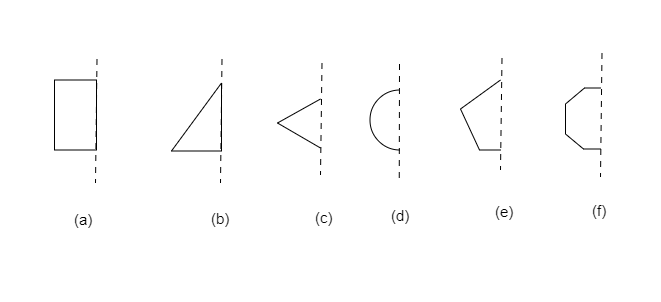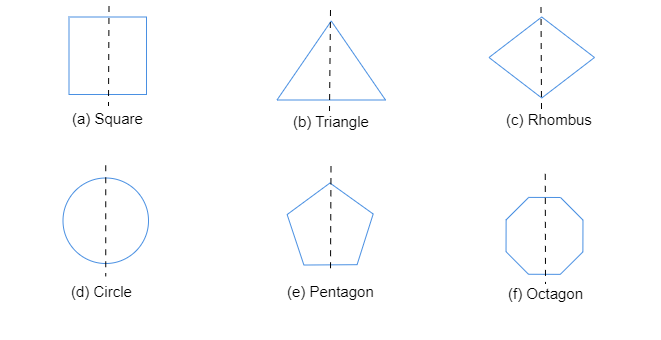"
">

# In the following figures, the mirror line (i.e., the line of symmetry) is given as a dotted line. Complete each figure performing reflection in the dotted (mirror) line. (You might perhaps place a mirror along the dotted line and look into the mirror for the image). Are you able to recall the name of the figure you complete?"

Given: In the given figures, the mirror line $(i.e.,\ the\ line\ of\ symmetry)$ is given as a dotted line.

To do: To complete each given figure performing reflection in the dotted (mirror) line. Also to name the figure completed

Solution:

We draw a line passing through the center point to each shape, in order to check their symmetry.$(a)$.The shape formed is a square.

$(b)$.The shape formed is a triangle.

$(c)$.The shape formed is a rhombus.

$(d)$.The shape formed is a circle.

$(e)$.The shape formed is a pentagon.

$(f)$.The shape formed is an octagon.

Updated on: 10-Oct-2022

40 Views